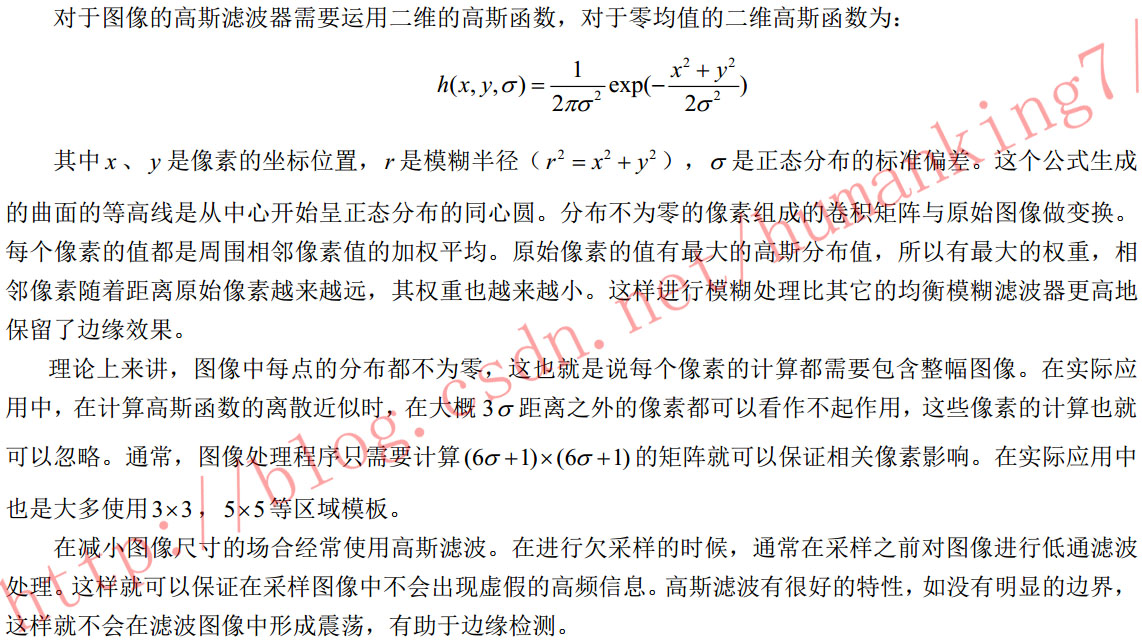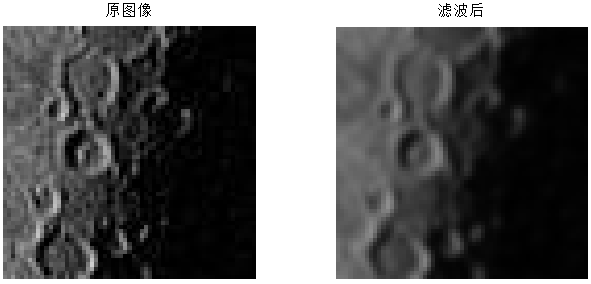• OpenCV二维高斯滤波核实现 matlab的fspecial函数，与matlab结果一致
//高斯核Mat gaussian_kernal(int kernelRowSize,int kernelColSize,double sigma0) { float halfRowSize = (kernelRowSize-1)/ 2.0 ;float halfColSize = (kernelColSize-1)/ 2.0; Mat K(kernelRowSize, kernelColSize, CV_32FC1); //生成二维高斯核 double s2 = 2.0 * sigma0 * sigma0;
for(float i = (-halfRowSize); i <= halfRowSize; i+=1) { int m = i + halfRowSize; for (float j = (-halfColSize); j <= halfColSize; j+=1) { int n = j + halfColSize; float v = exp(-(1.0*i*i + 1.0*j*j) / s2); K.ptr(m)[n] = v; } } Scalar all = sum(K); Mat gaussK;
K.convertTo(gaussK, CV_32FC1, (1/all)); return gaussK; }

展开全文opencv
• 最直接的方式就是使用MATLAB提供的函数imnoise(), 根据帮助文档中的调用格式 J = imnoise(I, ‘gaussian’, M, V)(M 为均值，V为方差)，想当然的将语句写为J = imnoise(I, ‘gaussian’, 0, 10^2)，但是运行后发现...
1.添加标准偏差为10的高斯噪声：imnoise
最直接的方式就是使用MATLAB提供的函数imnoise(), 根据帮助文档中的调用格式 J = imnoise(I, ‘gaussian’, M, V)(M 为均值，V为方差)，想当然的将语句写为J = imnoise(I, ‘gaussian’, 0, 10^2)，但是运行后发现完全不是预期的效果，因为加噪后的图像基本是一片白色，源图像几乎完全被淹没在噪声中。
在经过仔细阅读文档后发现，其实MATLAB的说明文档已经写得很清楚，现摘出如下：

J = imnoise(I,type,parameters) Depending on type, you can specify additional parameters to imnoise. All numerical parameters are normalized— they correspond to operations with images with intensities ranging from 0 to 1.

其中最关键的就是 normalized，即归一化，方差值在0~1之间。即默认的M，V值分别为0， 0.01（注意此处的方差形式）。
所以最终的结论就是 需要对方差归一化处理 ，比如此处要对一幅256*256的图像加入标准偏差为10的高斯噪声，那么相应的语句应为：
J = imnoise(I, 'gaussian', 0, 10^2/255^2)

2.设计高斯核算子：fspecial
f1 = fspecial('gaussian',h_size,σ) ;
//f1 = fspecial('gaussian',5*5,2) ;

σ越大或滤波器尺寸越大，图像越模糊
3.将算子与加入噪声的图像进行卷积imfilter
im1 = imfilter(IM,f1,'replicate');



展开全文• 1、二维高斯函数 G(x,y)=12πσ2exp⁡(−x2+y22σ2)G(x,y)=\frac{1}{2\pi {{\sigma }^{2}}}\exp \left( -\frac{{{x}^{2}}+{{y}^{2}}}{2{...3、Matlab实现 sigma1=2; k=5; % kernel大小 pai=3.1415926; kernel=zeros(k);
1、二维高斯函数
$G(x,y)=\frac{1}{2\pi {{\sigma }^{2}}}\exp \left( -\frac{{{x}^{2}}+{{y}^{2}}}{2{{\sigma }^{2}}} \right)$
2、坐标$(x,y)$（以5×5卷积核为例）3、Matlab实现
sigma1=2;
k=5;    % kernel大小
pai=3.1415926;
kernel=zeros(k);
m=(k+1)/2;
sigma=2*sigma1*sigma1;
for i=-1*(k-1)/2:(k-1)/2
for j=-1*(k-1)/2:(k-1)/2
kernel(i+m,j+m)=(-1/(pai*sigma))*exp(-1*(i^2+j^2)/(sigma));
end
end
kernel=kernel./sum(kernel,'all')  % 归一化

结果与Matlab函数fspecial()生成的高斯卷积核一致，卷积核如图所示：4、OpenCV实现
int main()
{
double sigma = 2, pai = 3.1415926;
int k = 5, m = (k + 1) / 2, n = m - 1;	// k-高斯卷积核大小
Mat kernel = Mat::zeros(Size(k, k), CV_64FC1);
double *p, Ksum;
sigma = 2 * sigma * sigma;
for (int i = -1 * n; i <= n; i++)
{
p = kernel.ptr<double>(i + n);
for (int j = -1 * n; j <= n; j++)
{
p[j + n] = (1 / (pai*sigma)) * exp(-1 * (i * i + j * j) / sigma);
}
}
Ksum = sum(kernel).val;
kernel = kernel.mul(1/Ksum);
cout << kernel << endl;

getchar();
return 0;
}



展开全文卷积
• ## [图像]高斯滤波(Matlab实现)

万次阅读 多人点赞 2015-07-10 09:16:55
核心提示 在Matlab高斯滤波非常方便，主要涉及到下面两个函数函数： fspecial 函数： imfilter 代码实现 clear all; clc; %---------------------------------------------- %对图像进行高斯滤波，并显示图像...
原创文章，欢迎转载。转载请注明：转载自 祥的博客

原文链接：http://blog.csdn.net/humanking7/article/details/46826105

简述

原理说明核心提示

在Matlab中高斯滤波非常方便，主要涉及到下面两个函数：

函数： fspecial
函数： imfilter
代码实现

clear all;
clc;
%----------------------------------------------
%对图像进行高斯滤波，并显示图像
%----------------------------------------------
%读进图像
[filename, pathname] = uigetfile({'*.jpg'; '*.bmp'; '*.gif'; '*.png' }, '选择图片');

%没有图像
if filename == 0
return;
end

Image = imread([pathname, filename]);
[m, n, z] = size(Image);

%转换为灰度图
if z>1
Image = rgb2gray(Image);
end

sigma = 1;
gausFilter = fspecial('gaussian', [5,5], sigma);
gaus= imfilter(Image, gausFilter, 'replicate');

%显示图像-----------------------
figure(1)
subplot(1,2,1);
imshow(Image);
title('原图像');
subplot(1,2,2);
imshow(gaus);
title('滤波后');

效果展示展开全文图像处理
• 函数实现了多元高斯核回归和平滑。 一些示例展示了如何使用它来平滑具有缺失数据的 3-d 噪声图像。 出于教程目的，该函数以最简单的形式实现。 因此，它特别适合初学者学习核回归。 更新版本通过搜索有效范围内...
• 非参数回归广泛应用于许多... 基于核密度估计技术，该代码实现了所谓的 Nadaraya-Watson 核回归算法，特别是使用高斯核。 回归的默认带宽来自文献中建议的高斯核密度估计的最佳弯曲宽度。 代码还可以处理丢失的数据。
• 高斯核分为水平方向和垂直方向，先后对图像进行卷积处理，可提高处理速度。 function output=gaussFilter(I,sigma) output=I; ksize=double(uint8(3*sigma)*2+1);%窗口大小一半为3*sigma window = fspecial('...
• 对于学图像专业的人来说，对图像进行高斯滤波应该不会陌生，本质上就是将图像...matlab中有函数能自动生成高斯卷积：  gs=fspecial('gaussian',3,1)  gs =  0.0751 0.1238 0.0751  0.1238 0.2042 0.1238
• mse matlab函数代码 ./experimental_files/: 仿真时用到的实验文件目录 ./gpml-matlab/: GPML库函数目录 ./data_read_and_MSE.m: ...目录下的代码，具体使用的核函数等参见data_read_and_MSE.m及实验报告
• 一篇15年文章的复原，先用kmeans均匀分块，然后高斯映射，然后meanshift精简。其中meanshift是固定阈值的，没加核函数
• 卷积其实是图像处理中最基本的操作，我们常见的一些算法比如：均值模糊、高斯模糊、锐化、Sobel、拉普拉斯、prewitt边缘检测等等一些和领域相关的算法，都可以通过卷积算法实现。只不过由于这些算法的卷积矩阵的特殊...
• 利用基于核函数的模糊均值聚类方法进行聚类分割图像。先求出隶属矩阵和聚类中心，通过高斯核函数计算样本点距离聚类中心的距离，若前后两次距离变化量小于某一阈值时算法终止，既得到聚类中心和隶属矩阵
• 需求说明：深度学习FPGA实现知识储备 ...卷积其实是图像处理中最基本的操作，我们常见的一些算法比如：均值模糊、高斯模糊、锐化、Sobel、拉普拉斯、prewitt边缘检测等等一些和领域相关的...
• （其中核函数选用高斯核） %run for parzen close all;clear all;clc; x=normrnd(0,1,1,10000);%从正态分布中产生样本 f=-5:0.01:5;%确定横坐标范 % N=100 h= 0.25 , 1, 4 p1=Parzen(x,0.25,10,f)...
• 简而言之，它使用混合了高斯的协方差核函数实现典型的高斯过程： k（t）= sum_ {q = 1} ^ Q w_q prod_ {p = 1} ^ P exp（-2pi ^ 2 t_p ^ 2 v_ {p，q} ^ 2）cos（2pi t_p m_ {p，q} ） 其中t = x-x'，q =混合物中Q...
• function C = SpectralClustering(data,k,a) %data是数据点矩阵 K是聚类个数 a代表高斯核函数的参数 %UNTITLED Summary of this function goes here % Detailed explanation goes here  d = pdis算法 function
• 高斯核 f(x,y,z) 因函数而异输入，请参阅下面的说明。 不提供图像填充，付费注意傅立叶环绕伪影。 部分支持各向异性平滑，各向异性体素大小为完全支持。 Suband_1.5 频率过采样用于减少当 sigma 小于体素长度时的...
• 平时，我们都是用matlab或者opencv的函数调用：imfilter或者cvSmooth，并不关心底层的实现。然而当开发者要实做高斯滤波的时候，往往就会很迷惘，往往会被以下几个问题困扰： 给定sigma，即标准偏差，怎么确定离散...
• 　二维高斯函数具有旋转对称性，处理后不会对哪一个方向上的边缘进行了过多的滤波，因此相对其他滤波器，具有无法比拟的优越性。但是传统Gauss滤波随着图像尺寸的增加，运算复杂度呈平方上涨，因此需要对其优化改进...
• 使用3D高斯核的半高全宽（FWHM）进行的空间低通滤波， 截止频率为1/128 Hz的瞬时高通滤波，可消除低频漂移。 电影统计（preprocessing_firstlevel.m） 在第一级设置感兴趣的t对比度 在每种情况下，随着棚车与血液...
• 高斯核（RBF-G-DF）的无散度RBF 具有多二次内核的3D RBF（RBF-MQ-3D） 具有多二次核的无散度RBF（RBF-MQ-DF） 3D B样条插值（SPL-3D） 具有拉普拉斯约束（SPL-LPL）的3D B样条插值 文件组织 data/包含这些实验生成的...
• Matlab实现的TTW，一种时间扭曲算法，它使用新的可变形卷积，移位Sinc来估计线性时间和空间复杂度中的DTW平均解。 这个仓库是什么？ 该存储库包含三种动态时间规整（DTW）平均算法的MATLAB代码：广义时间规整...
• 默认值=高斯核，即e -γ|| x-y || 2 ，宽度参数γ= 1。 accelerated_flag ：0或1，默认=0。如果该标志设置为1，则代码使用算法的加速版本，如的第5.2.1节所述。此版本将输出较低质量的Nyström近似值，但运行速度更...
• reference_opencv实现高斯核 reference_MATLAB_fspecial函数说明 # MATLAB H = fspecial('Gaussian', [r, c], sigma); # opencv-python # cv2.getGaussianKernel(r, sigma)返回一个shape为(r, 1)的np.ndarray, ...
• 简介 　Gabor变换属于加窗傅立叶变换，Gabor函数可以在频域不同尺度、不同...在空间域，二维Gabor滤波器是一个高斯核函数和正弦平面波的乘积，具体的：    复数：   实部：   虚部：      ...# 高斯核函数matlab实现matlab 订阅